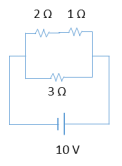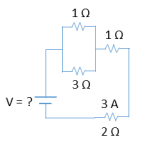Clutch Prep is now a part of Pearson
Ch 28: Resistors & DC CircuitsWorksheetSee all chapters

# Solving Resistor Circuits

See all sections
Sections
Intro to Current
Resistors and Ohm's Law
Power in Circuits
Microscopic View of Current
Combining Resistors in Series & Parallel
Kirchhoff's Junction Rule
Solving Resistor Circuits
Kirchhoff's Loop Rule

Concept #1: Solving Resistor Circuits

Transcript

Hey guys. So, in this video we're going to talk about how to solve more complicated circuit problems, let's check it out. Alright, so in some circuit problems you'll be asked to find not just the equivalent resistance but also the current i and the voltage V of different resistors. Alright, so let's see how we do that, but first, let me remind you that resistors can be connected in series or in parallel and if they're in series, this is the equation used to find the equivalent resistance, this is the equation for equivalent resistance in parallel. Remember, that in parallel there are also two shortcut equations you can use, two shortcuts, so this is old news, what's new here is this stuff here, right? And, when you have resistors in series they will share current with each other, they will share current with each other. So, for example, if I have a 1 ohm resistor 2 and 3, 2 ohms, 3 ohms and if I know that this current here is 2 amps then I know, if I'm given the current 2 amps then I know that this here has to be 2 amps and then I know that this here also has to be 2 amps, because they're in series, the current is flowing this way, it has nowhere to go so it has to just flow with a constant 2 amps. So, they share current with each other, cool? Now, you need to learn that but you also have to remember that they share current with the equivalent resistor as well So, they share current with the equivalent resistor, what does that mean? Well, if I combine these I just add them. So, I have one, two and three, this is 6 ohm resistor, but this 2 here is not only the same for these guys but it's also the same here. So, I'm going to know that this has to be a 2 amp current going through this resistor. So, not only is the current the same with each other when you're combining in series but also with the combined merged resistor, okay? Very important.

Practice: What is current and voltage across each resistor below?Example #1: Find Current of One Resistor

Transcript

Hey guys. So, this example I want to show you how some of these problems are actually deceivingly simple, they look complicated but they're very straightforward, this is an example of them. So, in this question instead of asking for everything, we're being asked for just one thing, which is what is the current through the swinging ohm resistor which is this guy here. So, that's all we need to know, let's call this the current through the 4 ohm, what is it okay? Well, remember, the equation that connects all these variables is Ohm's law V equals i, R. So, you can find i as long as you have V and R if you solve for i, u get V over R, I know the resistance is 3 ohms. So, really all I need is the voltage. Now, you may also remember that the voltage is the same in parallel and it's not just the same between resistors it's the same for any circuit, any circuit element, so the voltage across these two points is the same through that branch is the same as the voltage across these two points and it's the same for this voltage and it's the same for the voltage between these two resistors. So, because the voltage through this battery is 5, I can say that the voltage on the 3 ohms has to be 5 as well because they're parallel. So, that's it, this voltage is 5 volts, I can divide the two and the answer is going to be 1.67, 1.67 amps and we're done, super straightforward, but you have to be able to recognize certain properties, okay? So, some of these questions are going to be very long and some of them are going to be tricky and simple. So, you gotta be ready for both, let's keep going.

Practice: What is the voltage of the battery below?